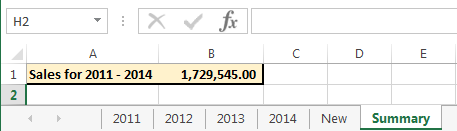# 3D Sum

In Excel, a 3D Reference refers to the same cell or range in multiple worksheets.  The 3D Sum allows you to sum the same cells in multiple worksheets.

To demonstrate this,  we have 4 worksheets and in Cell B1 of each sheet, there is a sales value that we want to get the total of.The summary sheet is where we want our total for all 4 years to appear.You are probably already familiar with adding cells across multiple sheets by typing “=” in the cell you wan the formula to appear in and then clicking on each cell in each sheet to be summed, with a “+” in between.An alternative way to do this is to use 3D Sum.

## 3D SUM

3D Sum is a  Sum formula

• Start it with “=SUM” and open bracket
• Click on Cell B1 of the first sheet (2011)
• Hold down shift and click on the last sheet (2014)
• Close the bracketsApart from how fast a 3D Sum can be completed, another advantage is that the formula will sum the Cell in every sheet within the range.

If we add a new sheet to anywhere between sheets 2011 and 2014 the formula will automatically include the cell of this sheet.

I’m adding a sheet called “New” and the value in B1 will be “8765432”

I will move this sheet between 2013 and 2014 and our formula result will updateIf this sheet was outside our selected range, the formula would not include it.If I was going to add a sheet called “2015” after the 2014 sheet, I could just edit the formula to include this sheet.

As useful and quick as 3D Sum can be, there are also potential drawbacks.

2 of the bigger potential issues I have come across include

1. The Cell being summed must be the same Cell reference across all sheets, in our case it is B1 above, but if the sheet layout changed then this may no longer be B1.
2. Other people – other people may change the sort order, add in sheets that fall within your selected sheet range, or do things to change the referenced cell or range of sheets.
[grwebform url=”http://app.getresponse.com/view_webform.js?wid=4307503&u=CucD” css=”on”/]